# College Chemistry : Nernst Equation

## Example Questions

### Example Question #6 : Redox Reactions

Consider the following redox reaction carried out in a voltaic cell.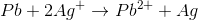If the reduction potential for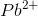is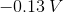and for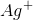it is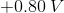, what is the value of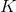and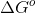for this reaction at a temperature of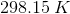?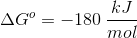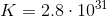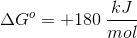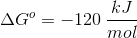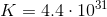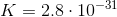Explanation:

For this question, we're given a redox reaction occurring within a voltaic cell. We're also supplied with the reduction potentials for the elements in the reaction, and are asked to find the standard free energy change as well as the equilibrium constant of the reaction at a given temperature.

Recall that in a voltaic cell, the reaction does not consume energy but rather produces it. The anode is where oxidation occurs, while the cathode is where reduction takes place. Based on the reaction given to us in the question stem, the anode will consist of solid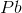, while the cathode will consist of solid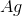. As oxidation occurs in the anode, the electrons liberated from the oxidation of solidwill result in the production of, which will exist as a cation in the aqueous solution. Moreover, these electrons will flow spontaneously from the anode to the cathode, releasing energy in the process. When the cathode receives these electrons, thein the aqueous solution will be reduced and thus deposited onto the cathode terminal as solid.

Knowing this, we can calculate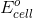for the reaction.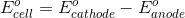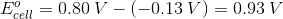Now that we havefor the reaction, we can plug this into the Nernst equation to solve for the equilibrium constant.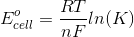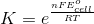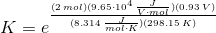A couple things to note. The value forused in the equation above isbecause this is the number of electrons transferred in the balanced reaction. Also, note that the value for this equilibrium expression is huge, meaning that the reaction is driven far to the right, making it spontaneous and capable of releasing a great deal of energy.

To find the value of, there are a few ways we can go about it. We can use the equilibrium value we just obtained, or we can use thevalue.

Using: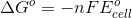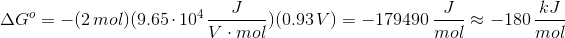Using: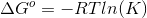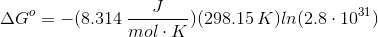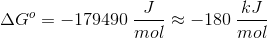As shown above, either method leads us to the same value for. And once again, note how large of a negative value this term is. This is consistent with the large value for the equilibrium constant. The reaction equilibrium lies far to the right and produces an enormous amount of energy.